Customize and Use Today!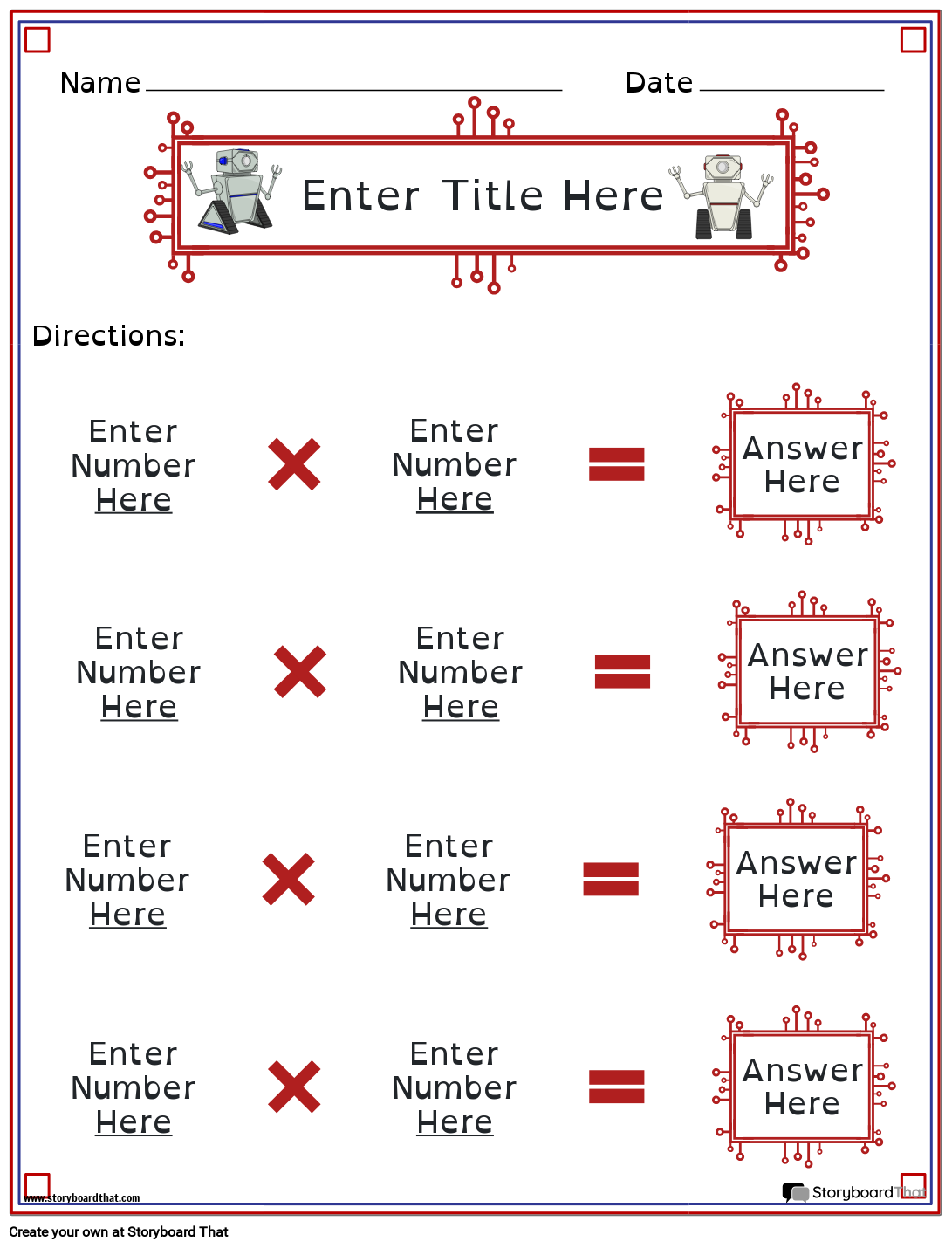Copy Template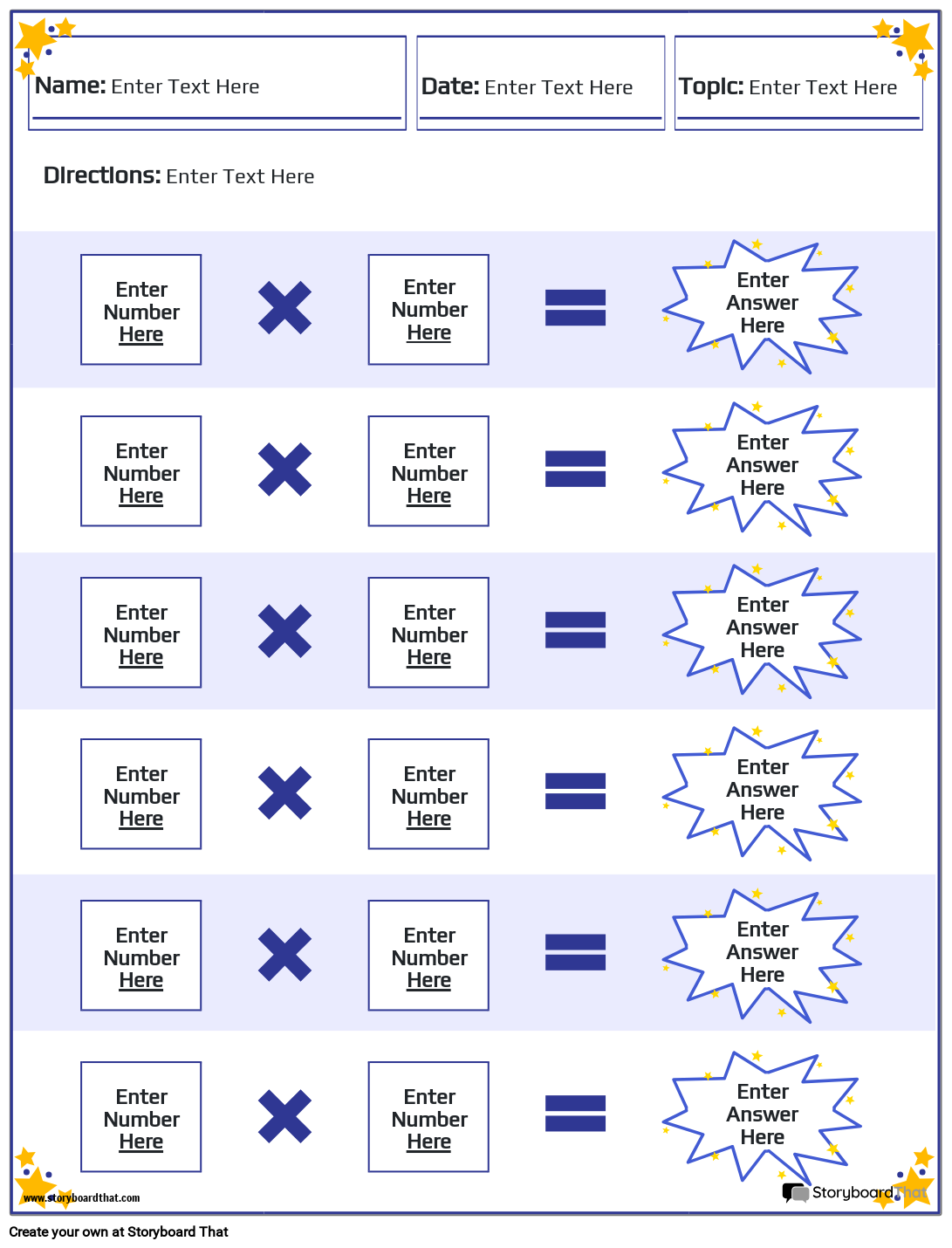Copy Template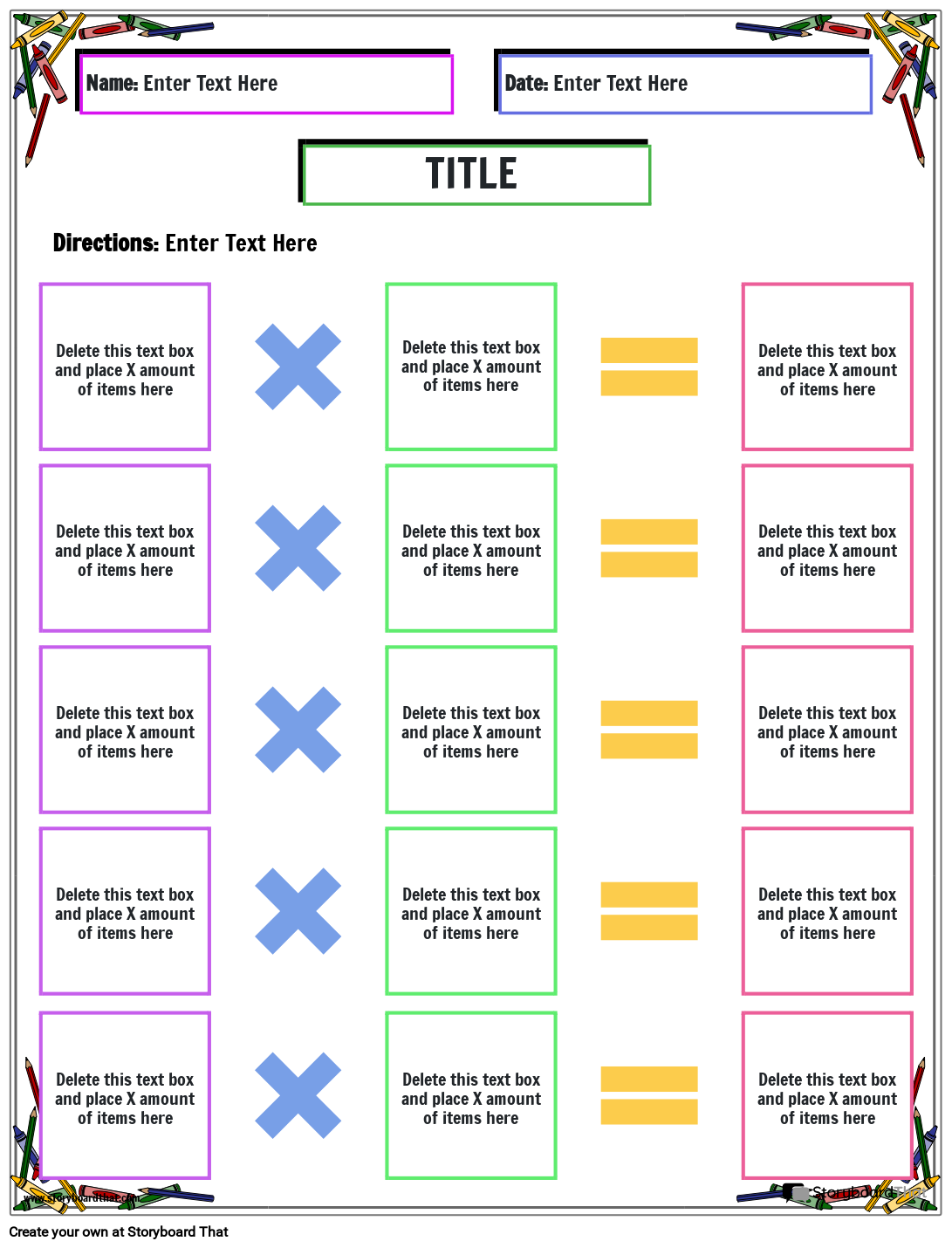Copy Template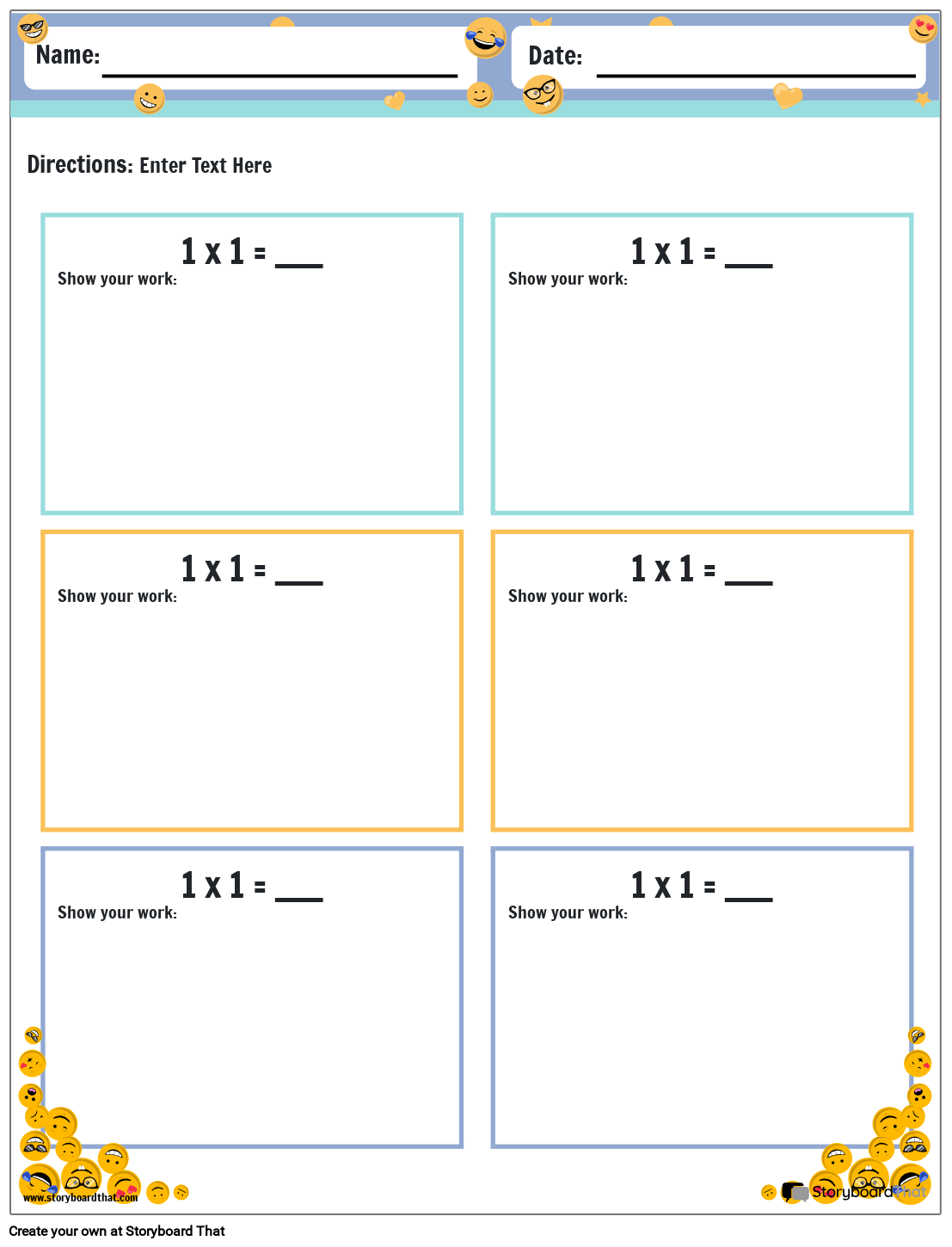Copy Template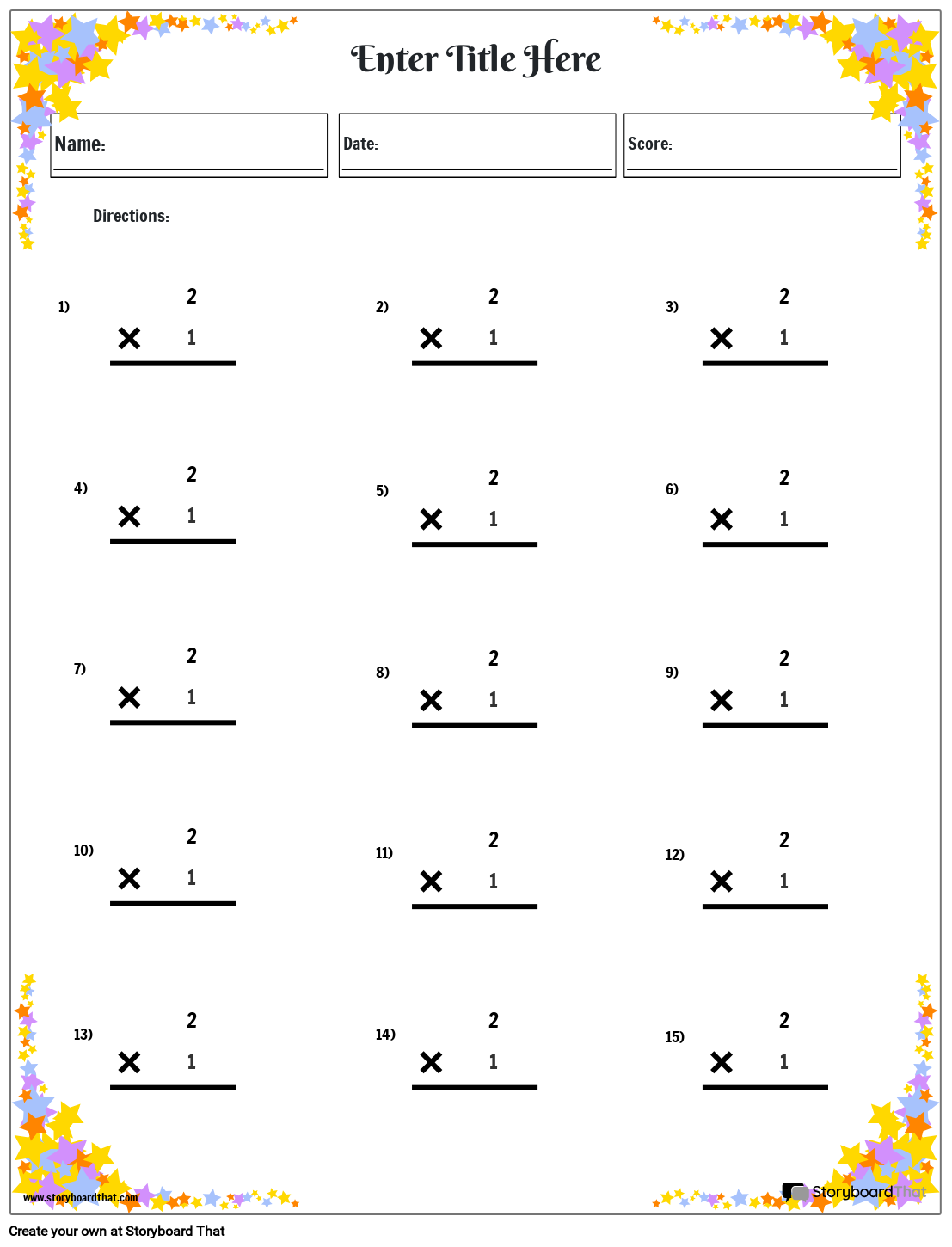Copy Template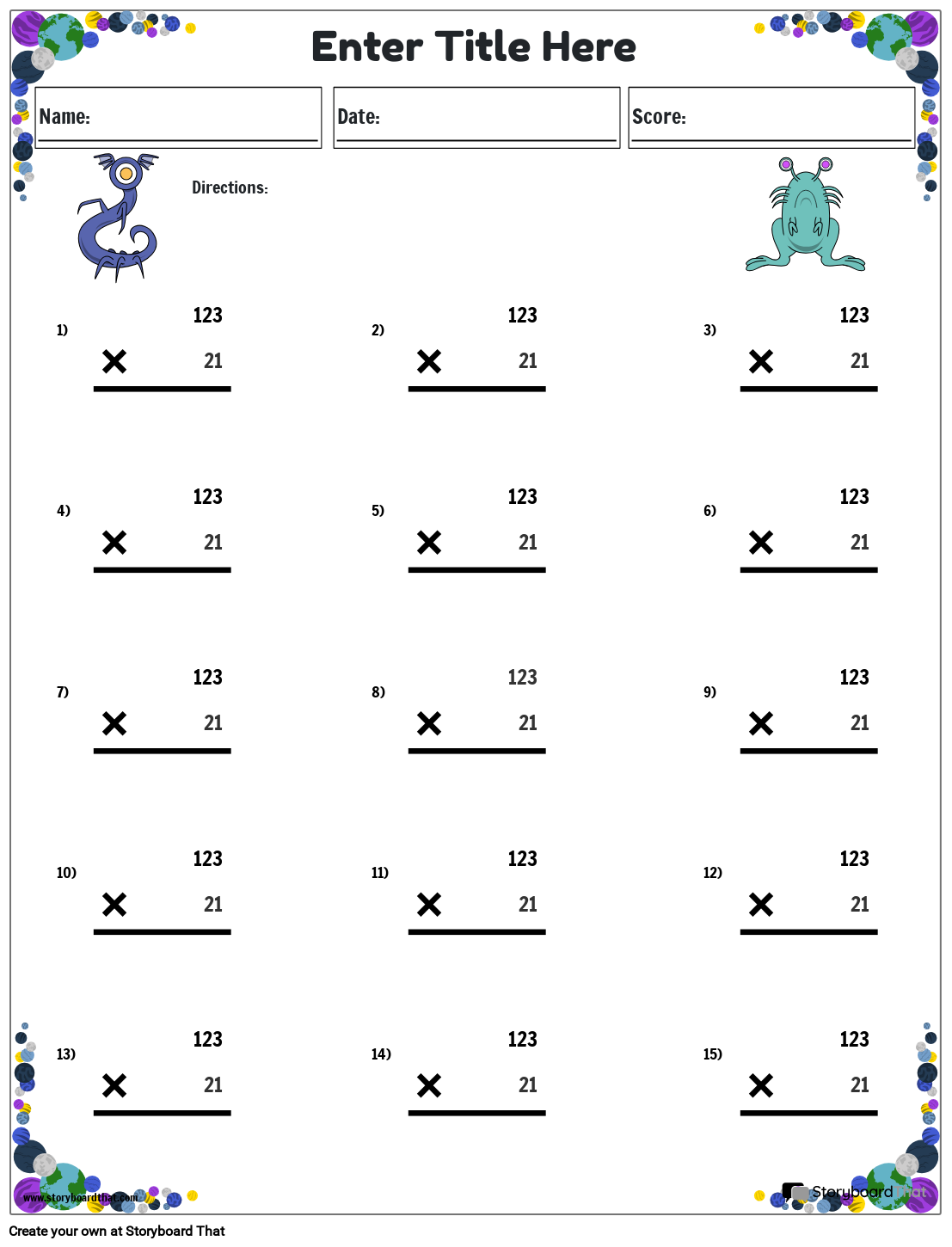Copy Template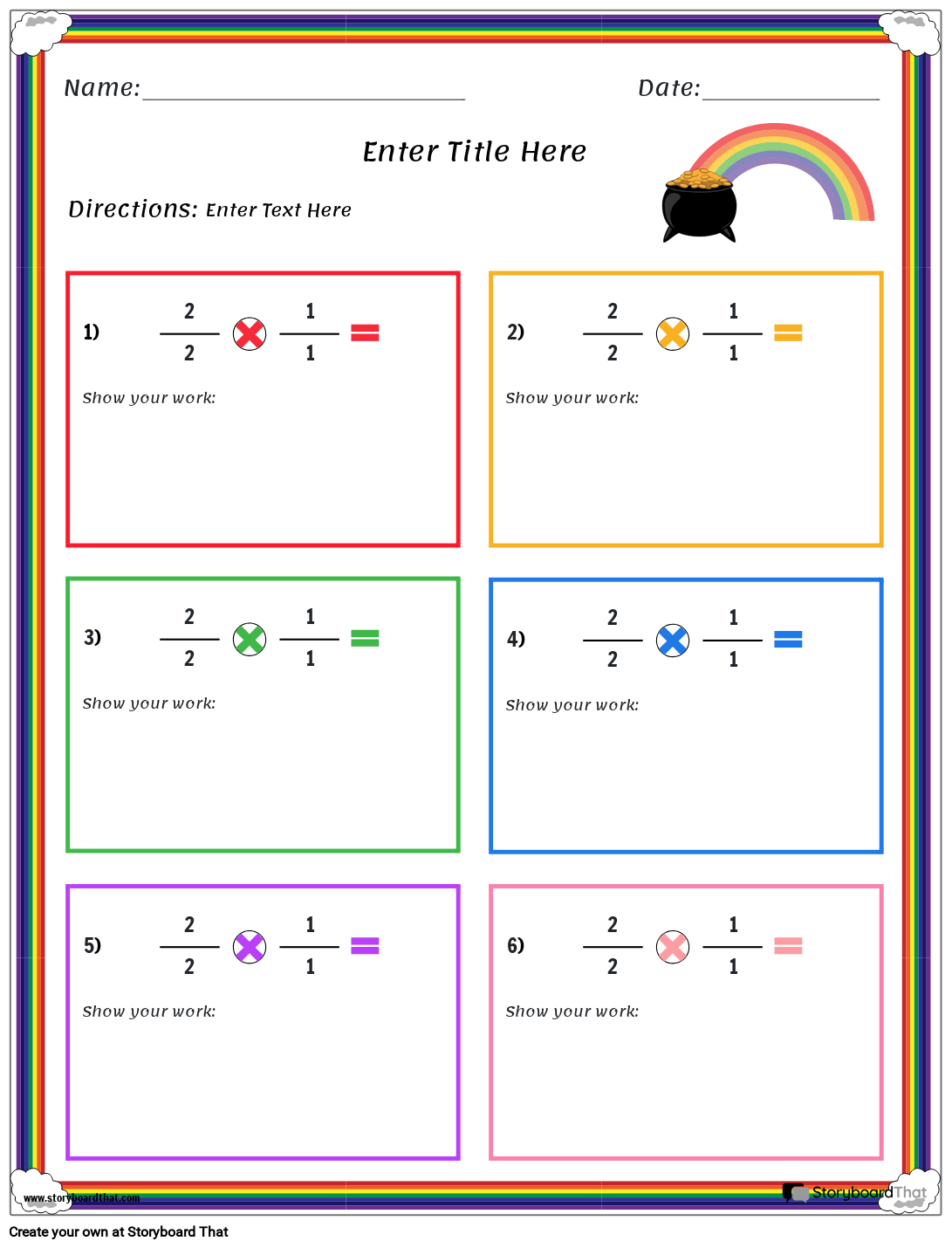Copy Template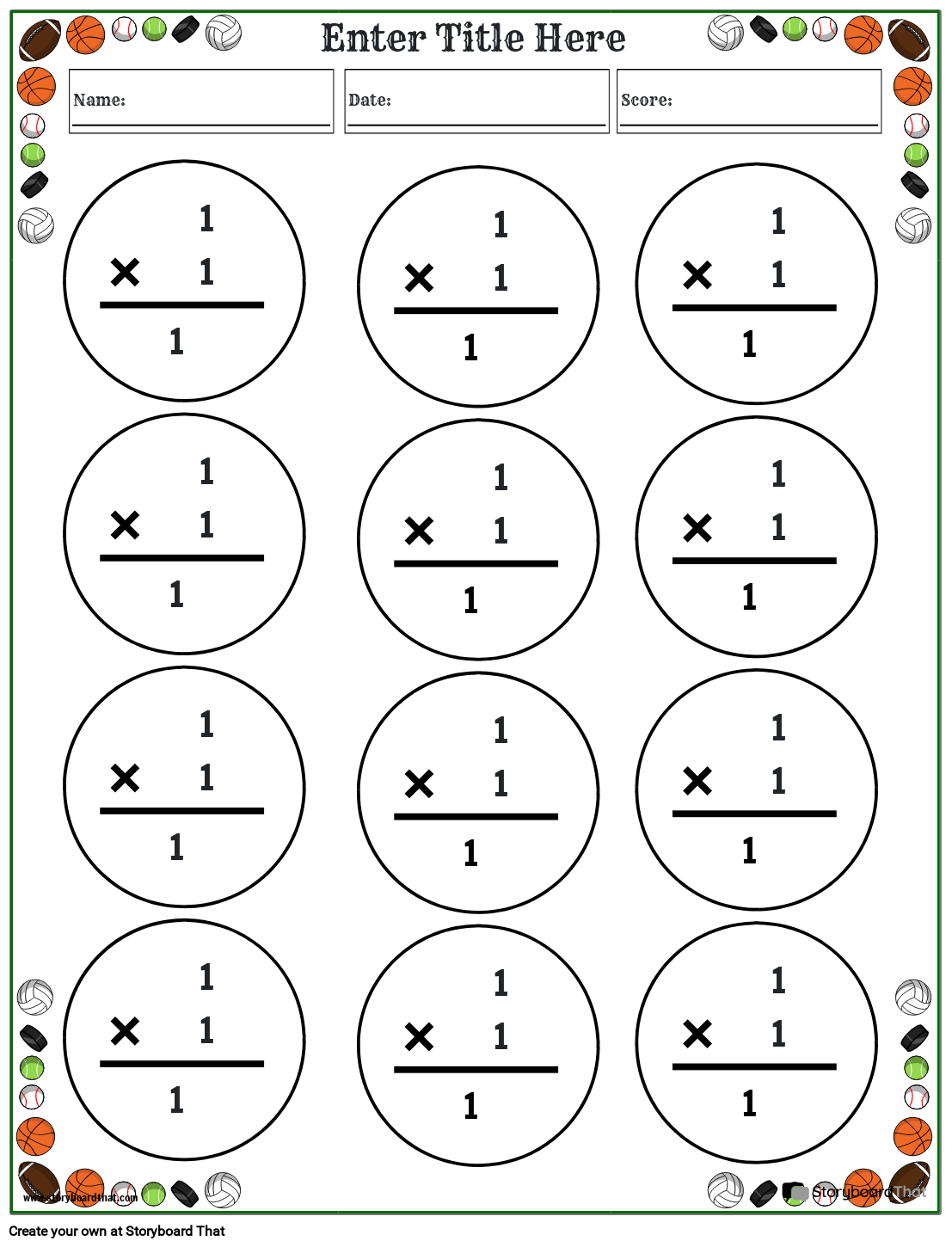Copy Template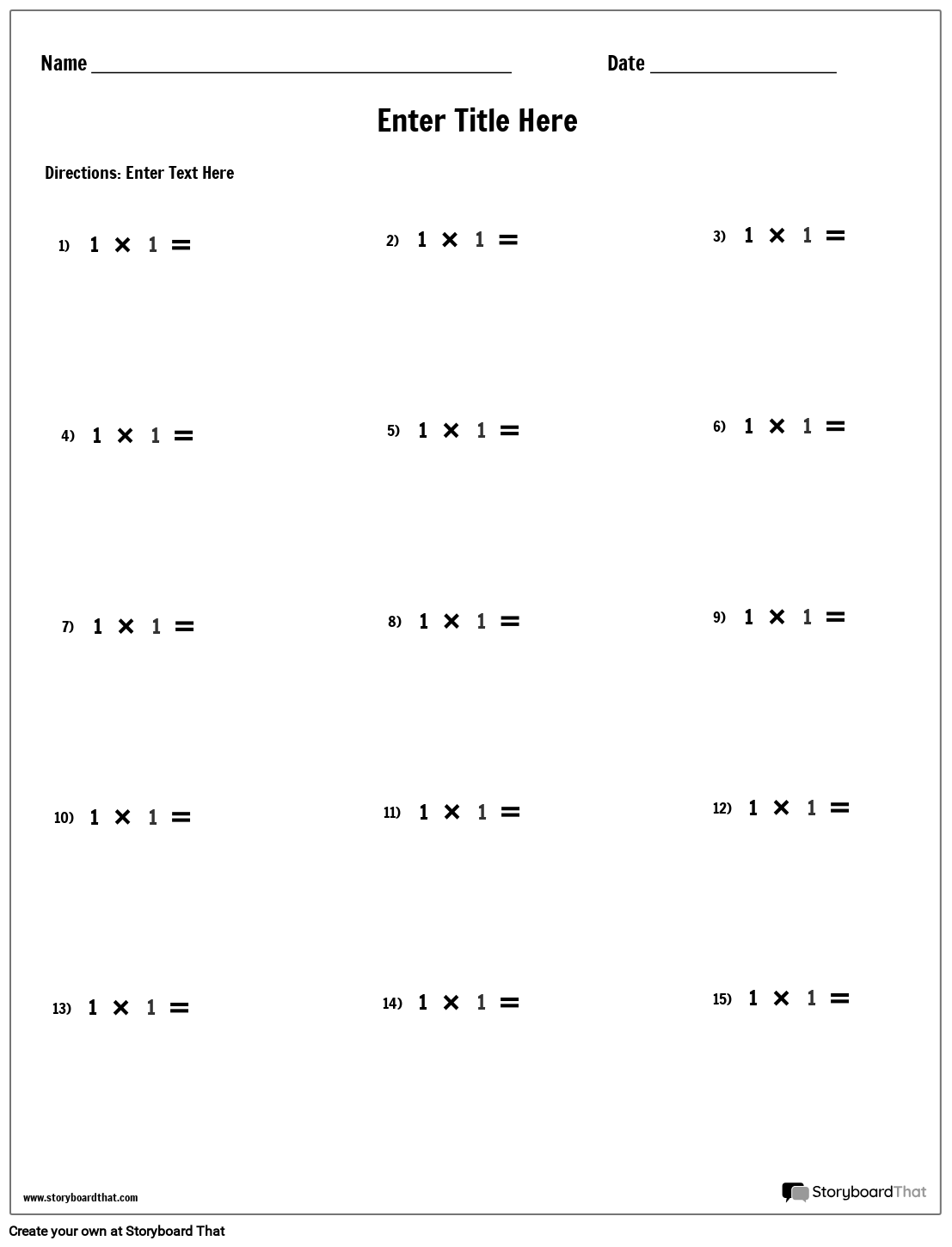Copy Template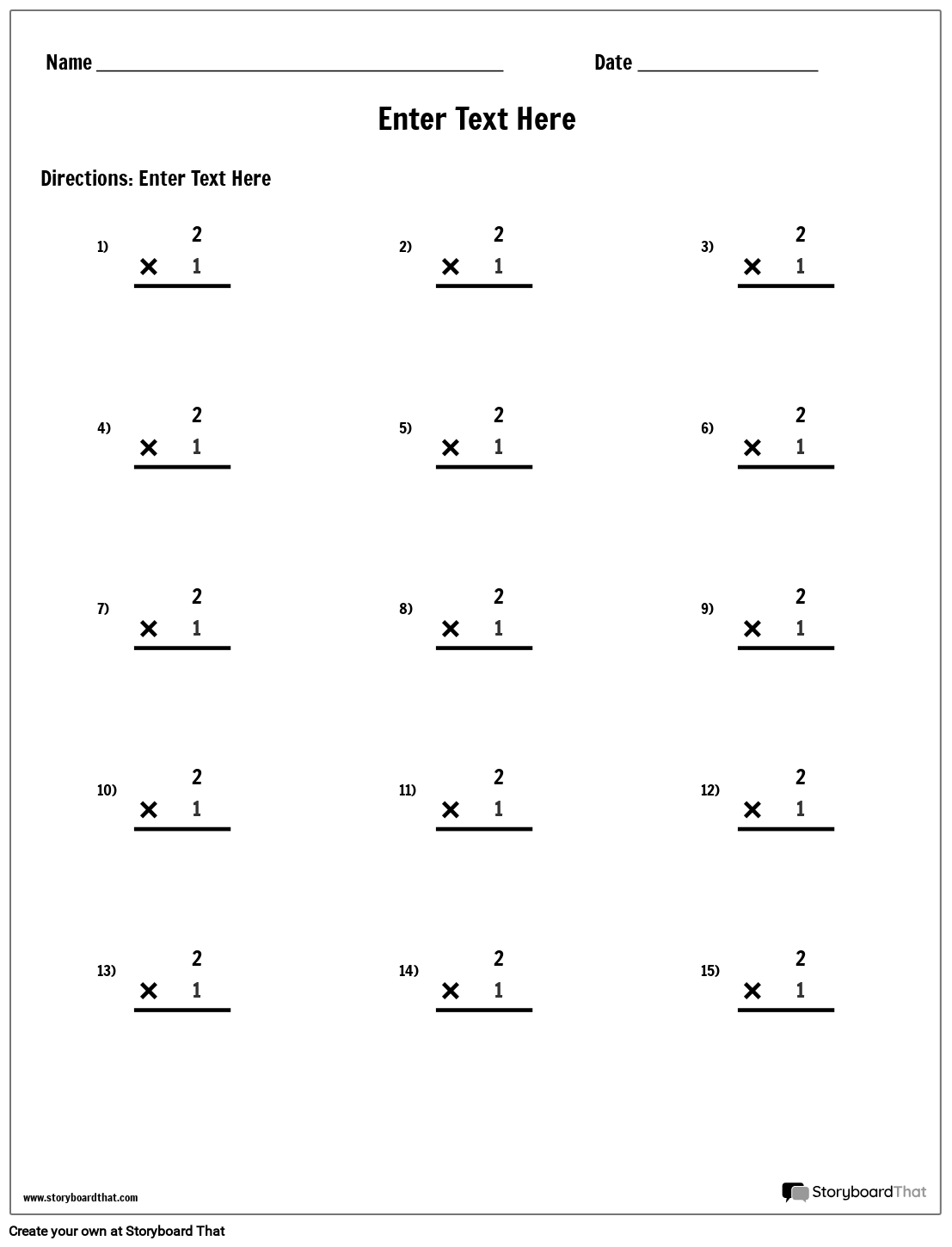Copy Template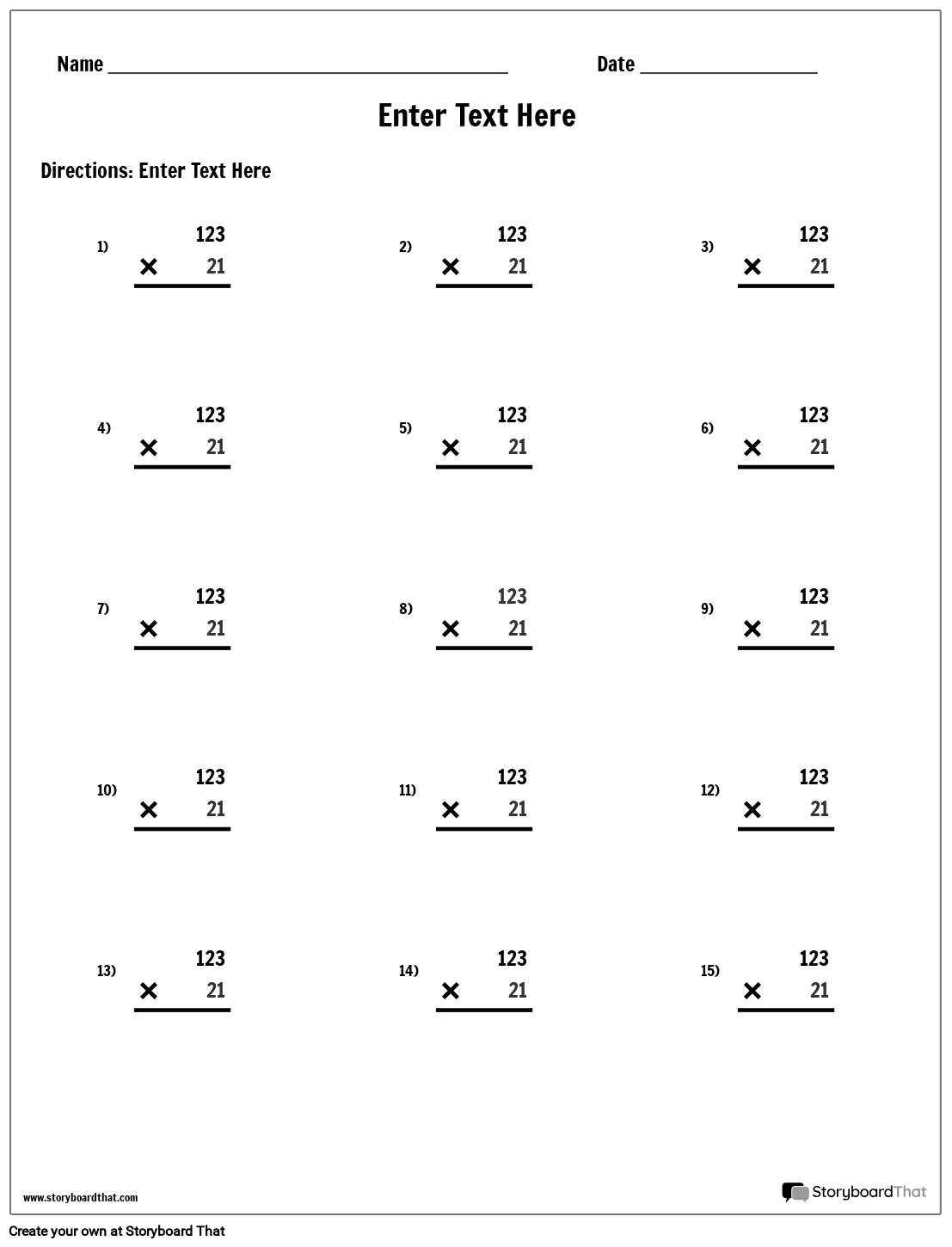Copy Template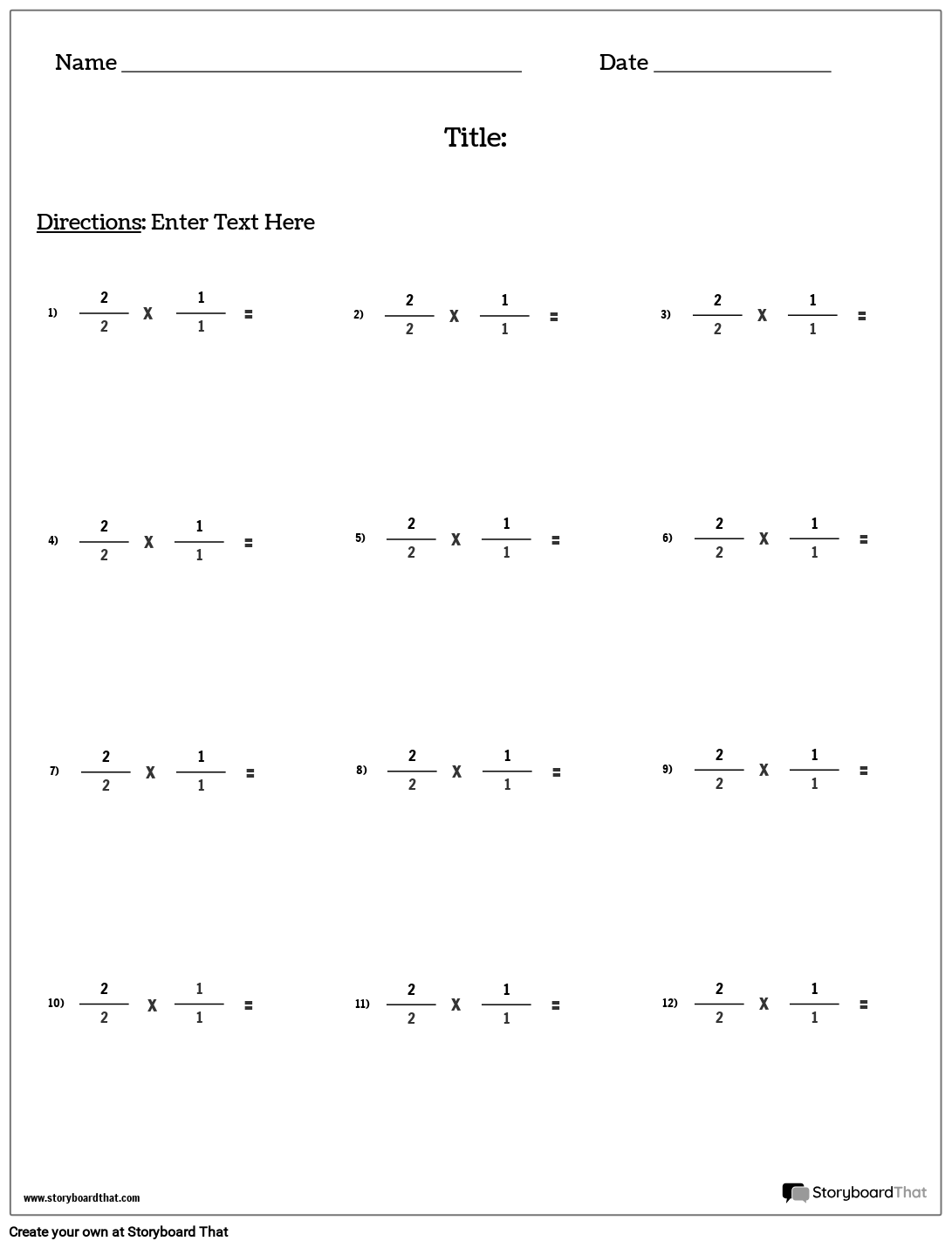Copy Template

If you're assigning this to your students, copy the worksheet to your account and save. When creating an assignment, just select it as a template!

## What are Multiplication Worksheets?

A multiplication worksheet is an educational material designed to help learners practice their skills. The multiplying worksheets typically include a series of problems that students must solve. The problems may be arranged in rows and columns, or they may be presented in a more random order.

Multiplication worksheets are also teacher tools that help kids learn the basic times tables, or to provide more advanced practice for those who are working on more complex problems and calculations. Some worksheets may also include word problems that require students to apply their multiplication skills in real-world situations.

Multiplying worksheets are commonly used in the classroom, but they can also be used at home for extra practice. They are available in a variety of formats, including printables that can be customized and downloaded, the worksheets also have high engagement or interaction available to be completed on a computer or mobile device.

## Why Are They Important and How Are They Best Used?

Multiplication worksheets are important because they provide students with an opportunity to practice and reinforce their multiplication skills. Regular practice can help develop fluency and accuracy, which is an essential skill for success in math and other subjects.

These tools are best used as a supplement to classroom instruction, rather than as a replacement for it. They can be used by teachers to provide additional practice for those who need extra support, or as a form of assessment to evaluate students’ understanding of the concepts. They can also be used as a multiplication tests sheet for students by the teacher.

To use the multiplication sheet effectively, it is important that students have a strong foundation in basic concepts before moving on to more advanced problems. Worksheets should be selected based on the needs and abilities of the individual, and should be appropriately challenging without being overwhelmed.

It is also important to provide feedback as they work on multiplication sheets. This can be done through individualized instruction, peer review, or automated feedback systems. Feedback helps students identify their strengths and weaknesses, and can motivate them to continue practicing and improving their multiplication skills.

## Multiplication Worksheet Templates

Multiplication worksheet templates are pre-designed documents that teachers or parents can use in creating their own customized multiplication worksheets. These templates typically include spaces for students to write their answers to the problems, as well as headings and formatting to make the worksheet clear and easy to use.

There are many different types of worksheets for available, depending on the needs of the user. Some common types:

1. Basic Multiplication Template: These templates include simple multiplication problems to solve, usually involving single digits numbers.
2. Word Problem Templates: These templates include word problems that require student to apply their multiplication skills in real-world situations.
3. Timed Multiplication Templates: These templates are designed to challenge kids to complete a set of multiplication problems within a certain time limit, in order to develop a speed multiplication worksheets with accuracy. There are some available games online that are timed multiplication for practice.
4. Multi-Digit Multiplication Template: These templates include more complex multiplication problems, involving two or more digits.

Multiplication worksheet templates are a convenient and effective tool for teachers and parents to use in helping students develop their mathematical skills.

## Math Worksheet Templates

There are various math worksheet templates available for teachers or parents to use to create customized math worksheets.

Here some common types of math worksheet templates:

• Addition and Subtraction Worksheet: These templates include spaces for students to solve addition and subtraction problems, ranging from simple single-digit problems to more complex multi-digit problems. The worksheet is a self taught tool that can be used to solve addition and subtraction problems.
• Multiplication and Division Worksheet: These templates are similar to addition and subtraction templates, but they focus multiplication and division problems instead. They may include tables or grids for students to use to solve problems.
• Fraction Worksheets: These templates focus on fractions, and may include a range of problems involving addition, subtraction, multiplication, and division of fractions.
• Geometry Worksheets: These templates include problems related to geometry concepts, such as angles, circles or shapes, area and perimeter, and measurement.
• Graphing Worksheets: these templates focus on graphing concepts, such as plotting points, drawing lines, and creating graphs.
• Time Math Worksheets: These templates are designed to challenge students to complete a set of math problems within a certain time limit, in order to develop speed and accuracy.

## Making Your Own Multiplication Worksheet

There are several ways to generate multiplication worksheets, but here is a simple method:

1. Determine the number of problems you want to include in the worksheet. For example, you may want to create a worksheet with 20 problems.
2. Decide on the range of numbers to be multiplied. For example, you may want to create problems that involve multiplying numbers 1 and 10.
3. Create a table with a word processor or spreadsheet software that has two columns. The first column should contain the multiplicand (the number being multiplied) and the second column should contain the multiplier (the number by which the multiplier is being divided).
4. Fill in the first column with the multiplicand you want to include in the worksheet. For example, if you want to include the numbers 1 through 10 as multiplicands, list them in the first column.
5. Fill in the second column with the multipliers. For example, if you want to include the numbers 1 through 10 as multiplicands, list them in the second column.
6. Multiply each pair of numbers in the table to create the problems. For example, for the first row of the table, the problem would be 1 x 1 = ____, and for the second row, the problem will be 1 x 2 = _____.
7. Add a blank space or line for kids to write their answers on the paper or sheet.
8. Format the worksheet as desired, including any instructions or additional information, and it can be printed out for distribution to students.

Creating a worksheet from scratch is fun, but you can also save lots of time with our premade templates! Check out how to copy a template below.

## How to Create a Multiplication Table

1

### Choose One of the Premade Templates

We have color and black and white portrait templates. Take a look at our example for inspiration!

2

### Click on "Copy Template"

Once you do this, you will be directed to the storyboard creator.

3

### Give Your Worksheet a Name!

Be sure to call it something related to the topic so that you can easily find it in the future.

4

This is where you will include directions, specific questions and images, and make any aesthetic changes that you would like. The options are endless!

5

### Click "Save and Exit"

When you are finished with your worksheet, click this button in the lower right hand corner to exit your storyboard.

6

### Next Steps

From here you can print, download as a PDF, attach it to an assignment and use it digitally, and more!

## Other Resources to Use!

Here are the few other templates to use with your class:

• Division Worksheet Templates: Division worksheet templates are pre-designed worksheets that provide structured formats for practicing division problems. These templates typically include a series of math problems that are organized in rows and columns. The problems may be arranged in various ways, such as horizontally or vertically, and may include a mix of single-digit, double digit, or larger numbers.
• Blank Worksheet Templates: Blank worksheet templates are pre-designed that have no content or problems in them, allowing users to customize them to their specific needs, especially a color worksheet. The blank worksheet templates also provide basic structure for creating various types of worksheet, including math problems, vocabulary exercises, writing prompts, and more.
• Addition Worksheet: An addition worksheet is a type of worksheet used to practice and reinforce addition skills. Addition is a fundamental mathematical operation that involves combining two or more numbers to get a total or sum.

Happy Creating!

### What kind of problems are typically included on a multiplication worksheet?

Multiplication worksheets can include a wide range of problems, from simple one-digit problems to more complex multi-digit questions. The multiplication worksheet is also a type of math worksheet that involves different types of numbers, such as whole numbers, decimals, and fractions.

### Are there any resources available to help me create a multiplication worksheet?

Yes, there are many resources available on Storyboard That that help you create multiplication worksheets, such as worksheet generators and templates.

### How can I differentiate worksheets for students with different skill levels?

To differentiate the multiplication sheet, you can adjust the difficulty level of the problem based on each student’s skill level. For example, you can provide simpler problems for students who just started learning multiplication, and more challenging problems for advanced students. You can also provide images, such as arrays and diagrams, to help students understand the concepts behind multiplication.

### How often should I use multiplication worksheets in my classroom?

The frequency of using multiplication worksheets in your classroom will depend on your teaching style and curriculum. However, it is generally recommended to incorporate regular practice of skills throughout the school year to help students build and reinforce their understanding.

View all Worksheet Templates!
View All Teacher Resources
*(This Will Start a 2-Week Free Trial - No Credit Card Needed)